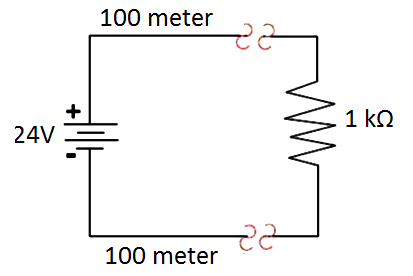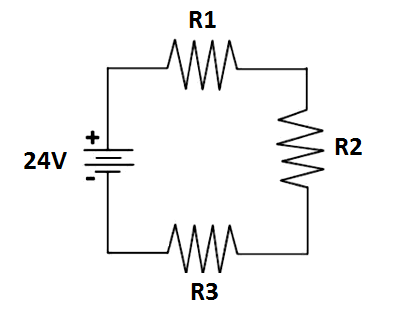Home » Instrument Power Cable Sizing Details
Instrumentation Design

Instrument Power Cable Sizing Details

Instrument power cable sizing is a calculation of voltage drop across some distance from instrument marshalling cabinet to field devices. It is needed to make sure that the supply voltage at the field devices is sufficient (within device operating voltage or within specified requirement).

Power Cable Sizing

For example, we have a solenoid valve put at 250 meter from marshalling cabinet. This solenoid valve requires a supply voltage 24 VDC. Due to a long distance separated, the voltage received at solenoid valve will not be 24 VDC. It may be 23.5 VDC, 22 VDC, or even 15 VDC. It depends on the size of cables that used.

So, the purpose of the instrument power cable sizing is to select the appropriate cable size so that the voltage drop received at field devices is within an acceptable range or within specified requirements. So, how to perform such calculation?

First of all, let’s take a look at below circuit.This schematic is an illustration of equipment that has 1 K OHM resistance and separated far away from the power supply by 100 meter. Let’s assume that the cable has an inherent resistance 1 OHM/meter so that in this case, the resistance from power supply to equipment will be 100 OHM.

We can replace the above schematic with below simplified schematic to calculate the voltage drop received at equipment.Where,

V = 24 Volt

R1 = 100 OHM

R2 = 1K OHM

R3 = 100 OHM

Now we could look for the equivalent replacement resistor of this 3 resistor.

RTOT = R1 + R2 + R3

RTOT = (100 + 1000 + 100)  OHM

RTOT = 1200  OHM

After we calculate the equivalent replacement resistor (RTOT) now we could get the current that flow through this circuit.

I = V / RTOT

I = 24 / 1200

I = 0.02 A

Then we shall go back to above circuit to find the voltage received by the R2 (which is our equipment).

VR2 = I * R2

VR2 = 0.02 * 1000

VR2 = 20 Volt

From above calculation we could determine that the voltage drop is 4 volt. Each drop is contributed by R1 and R2 as follows:

VR1 = VR3 = I * 100 = 0.02 * 100 = 2 Volt.

Each resistor (R1 & R3) contribute 2 volt voltage drop.

At actual application we rarely know the resistance of our equipment. What usually specified by manufacturer is only a power consumption at nominal power supply (can be 24 VDC or 48 VDC).

So, how to get the equation to calculate the voltage drop across some distance with using the power consumption data?

From previous illustration in the part 1, we could express the voltage drop (Delta V) as below:

Delta V = V – VR2

Delta V = V – I*R2

Because I=V/RTOT then,

Delta V = V – ( (V/RTOT) * R2)

We take out the same V so that we could get the following equation,

Delta V = V* (1 – (R2/RTOT) )

Since the RTOT = R1+R2+R3 then,

Delta V = V* (1 – (R2 / (R1+R2+R3) ) )

Since R1 = R2 = Length of cable (L) * Resistance of cable (Rcable) then,

Delta V = V* (1 – (R2 / ((L*Rcable) + R2 + (L*Rcable)) )

Delta V = V* (1 – (R2 / ((2*L*Rcable) + R2)

Unfortunately we don’t know the R2 of the equipment (but we only know the power consumption), then we should replace this in term of power consumption (P).

R2 = P/I2

While I = P/V, then

R2 = P / (P/V)2

R2 = V2 / P

Now we substitute this R2 value to voltage drop equation above.

Delta V = V* (1 – ( (V2 / P) / ((2*L*Rcable) + (V2 / P) )

Delta V = V* ( ((2*L*Rcable) + (V2/P) – (V2/P)) / (2*L*Rcable) + (V2/P) )

Delta V = V* ((2*L*Rcable) / (2*L*Rcable) + (V2/P))

While (2*L*Rcable) + (V2/P) = (2*L*Rcable) + R2 = RTOT

Then we could simplify the above equation to be:

Delta V = V/RTOT * (2*L*Rcable)

Delta V = I * (2*L*Rcable)

Where,

Delta V = Voltage drop across some distance (Volt)

I = electric current (Ampere) = P/V

L = Length of cable (km or m)

Rcable =  OHM/km or  OHM/m

Usually Rcable represents in OHM/km rather than in OHM/m. And length of cable represents in m. In this case the voltage drop equation will be:

Delta V = (I * 2*L*Rcable) / 1000

Let’s take some example.

We have a solenoid valve put at distance 250 meter from the system cabinet (power supply putted). This solenoid valve has a power consumption 5 [email protected] VDC. We need to check what cable size that can be used with the requirement of voltage drop must not exceed 5% at solenoid valve terminal. The following example cable data will be used:

The calculation:

Calculate the electric current:

I = P/V = 5 watt / 24 V = 0.2083 A

Calculate the voltage drop by using formula:

Delta V = (I * 2*L*Rcable) / 1000 with the preliminary Rcable using the 1.0 mmsq properties.

Delta V = (0.2083*2*250*20)/1000

Delta V = 2.083 Volt

Delta V = 2.083 Volt / 24 Volt *100%

Delta V = 8.67%

It exceeds our requirement which is 5%. So using 1.0 mmsq cable isn’t sufficient for our application. Let’s try with 1.5 mmsq cable.

Delta V = (0.2083*2*250*15)/1000

Delta V = 1.56 Volt

Delta V = 1.56Volt / 24 Volt *100%

Delta V = 6.5%

By using 1.5 mmsq cables it still exceed the requirement. Let’s try again with 2.5 mmsq cable, looks like this cable will suit for this application.

Delta V = (0.2083*2*250*10)/1000

Delta V = 1.04 Volt

Delta V = 1.04Volt / 24 Volt *100%

Delta V = 4.34% which is less than 5% requirement.

Yes, this 2.5 mmsq cable is suitable for our applications. Thus this cable size will be selected for this application.

Articles You May Like :

How to Select Flow Meter

What is a Four-wire RTD ?

Thermocouples and RTD’s

Freewheeling Diode Principle

Switch or Transmitter

Why we use Cable Shielding with Ferrites ?

This website uses cookies to improve your experience. We'll assume you're ok with this, but you can opt-out if you wish. Accept Read More

WordPress Image Lightbox

Send this to a friend